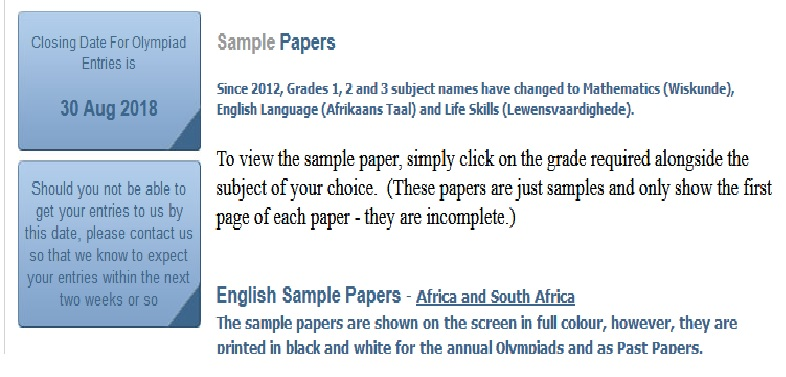1. Home
2. »
3. Question Paper
4. »
5. Conquesta Olympiads Mathematics 1 Sample Ques...
All posts from

Name of the Organization : Conquesta Olympiads
Announcement : Conquesta Olympiads Mathematics 1 Sample Question Paper
Type : Sample Question Paper

Want to comment on this post?

### Instructions

** When you have decided which of the answers is correct, scratch out the letter in the matching square on your answer sheet using ONLY a black or blue ballpoint or black khoki pen.

Related : National Senior Certificate Exam NSC Sample Question Paper : www.southafricain.com/1696.html

** Do not use pencils, crayons, pencil crayons, highlighters, tippex or glue.
** If the answer to question 4 is c, then scratch out the letter c in the square containing c next to the number 4 (see example 1 below).
** If you’ve made a mistake and b should have been the answer, neatly cross out the mistake and then scratch out bUseful tip :
When you have number sentences using different operations, apply the rule of BODMAS, which is the order of operations:
Firstly, calculate whatever is in Brackets, then Other (of, square roots, power of, etc), then Division and Multiplication (from left to right as they rank equally), and lastly, ddition and Subtraction (also from left to right).

### Sample Questions

1. Three hundred and twenty million, four hundred and six thousand, three hundred and one, can be written as ”
(a) 3 246 301
(b) 32 460 301
(c) 300 264 631
(d) 320 406 301

2. 2. In the number 27 999 199 what is the value of the nine which is three places from the left of the number?
(a) ninety thousand
(b) ninety-nine thousand
(c) nine hundred thousand
(d) nine hundred

3. What is 10 less than one million?
(a) 999 990
(b) 199 000
(c) 90 000
(d) 999 000

4. Which one of the following becomes 474 000, when rounded off to the nearest thousand?
(a) 473 059
(b) 473 590
(c) 473 390
(d) 474 530

5. 12 000 ÷ 400 is the same as ”
(a) 1200 ÷ 40 ÷ 20
(b) 12 000 ÷ 20 ÷ 100
(c) 12 000 ÷ 2 ÷ 100
(d) 12 000 ÷ 2 ÷ 200

6. 600 000 ÷ 300 = ” x 100
(a) 2
(b) 20
(c) 200
(d) 2 000

7. 625 – 5 x 51 + 39 = ” (Apply the BODMAS rule.)
(a) 31 659
(b) 55 800
(c) 409
(d) 175

8. (250 + 45 x 2) x 12 + 8 – 13 x 2 = ” (BODMAS rule)
(a) 14 150
(b) 7 062
(c) 5 874
(d) 4 062

9. 930 girls and boys were divided into 30 teams. In each of the teams there were 21 girls. How many boys were there altogether?
(a) 200
(b) 300
(c) 270
(d) 321

10. The diagram shows the front view of a house of cards of 3 storeys high.How many cards are needed for a house of 10 storeys?
(a) 155
(b) 165
(c) 100
(d) 135

11. What are the next two numbers in this sequence?
-23, -17, -11 ,……
(a) -5, -1
(b) +5, -1
(c) -5, -2
(d) -5, +1

Match the mathematical terms in (a) – (d) with the definitions given in questions 12–15.
(a) equation
(b) ratio
(c) formula
(d) frequency

12. The use of letters or words to give a rule.
13. The number of times that something happens.
14. Where symbols and letters are used instead of numbers.
15. The comparison of one amount to another.

16. The high temperature in the Antarctic on one day was -42 degrees. The low on the same day was -62 degrees. What was the difference between the two?
(a) -20 degrees
(b) -109 degrees
(c) +20 degrees
(d) +109 degrees

Mathematics 1 – Grade 6 :
1. How should the following expanded notation be written in figures?
(8 x 103) + (5 x 105) + (10 x 10²) + (3 x 101)
(a) 580 103
(b) 508 130
(c) 590 300
(d) 509 030

2. Choose the correct sign(s) to go into the space.
(7 x 5) – 4 7 + (5 – 4)
(a) >,
(b) <,
(c) =
(d) None

I am a 3-digit number. My last digit is double my middle digit and 4 times my 1st digit. My 1st digit is a prime number.
3. What number am I?
(a) 312
(b) 248
(c) 520
(d) 224

4. Which two signs are missing from the following equation?
88 4 = 2 11
(a) x, +
(b) ÷, x
(c) +, x
(d) ÷, +

5. 26 + ½ of 8 x 4 = 66. (Apply BODMAS Rule.)
(a) 848
(b) 120
(c) 168
(d) 42

Mathematics 1 – Grade 5 :
1. What is the value of the underlined digit?
79 602 117
(a) 900 000
(b) 1 million
(c) 9 million
(d) 900 thousand

2. What is (( 2 + 900 + 3000 + 50 000 = ?
(a) 50 392
(b) 53 902
(c) 52 920
(d) 53 920

3. Find the missing number.
? + 20 = 4 010
(a) 3 090
(b) 3 980
(c) 3 080
(d) 3 990

4. Which number, when rounded off to the nearest hundred, gives 43 300?
(a) 42 935
(b) 43 249
(c) 43 294
(d) 43 408

5. How should eight ones, nine thousands, two hundred thousands, four hundreds and five tens be written as a number?
(a) 89 245
(b) 209 458
(c) 259 408
(d) 892 450

1.2.